# Math Grade 11 Algebra 2

Sale ends in
Teacher: Catherine
Customers Who Have Viewed This Course: 1706
\$280.00
\$117.00

#### 0 Course Introduction 02:07This course includes:

66 video lessons

This course introduces material found in a typical Algebra 2 class including the following topics:

Unit 1: Inequalities, Absolute Value Equations, Absolute Value Inequalities, General Problem Solving

Unit 2: Graphing Parent Functions and Transformations, Graph Reading, Graphing Piecewise Functions, Functions, Composite Functions, Inverse Functions

Unit 3: Solving systems in 2 variables, Solving systems in 3 variables, Systems of Inequalities, Linear Programming

Unit 4: Basic Matrices, Multiplying Matrices, Determinants, Inverse Matrices, Matrix Equations

Unit 7: Definition of Logarithms, Properties of Logarithms, Common Logarithms, Natural Logarithms, Change of Base, Logarithmic Equations, Graphing Logarithms

Unit 8: Graphing Polynomials, Long Division of Polynomials, Synthetic Division of Polynomials, Zeros of a Polynomial

Unit 9: Simplify Rational Expressions, Multiple/Divide Rational Expressions, Add/Subtract Rational Expressions, Complex Fractions, Rational Equations, Graphing Rational Functions

Unit 10: Circles, Parabolas, Ellipses, Hyperbolas

Unit 11: Sequences, Series

#### 1 Lesson 1 - Inequalities 19:57

In this lesson we discuss the intersection and union of sets and learn basic and compound inequalities with real world application.

#### 2 Lesson 1 - Inequalities Answer Key 20:35

Lesson 1 - Inequalities Answer Key

#### 3 Lesson 1 - Inequalities Quiz 10:00

Lesson 1 - Inequalities Quiz

#### 4 Lesson 2 - Absolute Value Equations 12:13

In this lesson we will learn how to solve an absolute value equation.

#### 5 Lesson 2 - Absolute Value Equations Answer Key 11:51

Lesson 2 - Absolute Value Equations Answer Key

#### 6 Lesson 2 - Absolute Value Equations Quiz 10:00

Lesson 2 - Absolute Value Equations Quiz

#### 7 Lesson 3 - Absolute Value Inequalities 11:54

In this lesson we will learn how to solve absolute value inequalities.

#### 8 Lesson 3 - Absolute Value Inequalities Answer Key 07:52

Lesson 3 - Absolute Value Inequalities Answer Key

#### 9 Lesson 3 - Absolute Value Inequalities Quiz 10:00

Lesson 3 - Absolute Value Inequalities Quiz

#### 10 Lesson 4 - General Problem Solving 22:00

In this lesson we will go through general problem solving techniques with an emphasis on number sense, literal equations, distance rate time problems, mixture problems, compound interest problems, and work problems.

#### 11 Lesson 4 - General Problem Solving Answer Key 1 15:38

Lesson 4 - General Problem Solving Answer Key 1

#### 12 Lesson 4 - General Problem Solving Answer Key 2 18:45

Lesson 4 - General Problem Solving Answer Key 2

#### 13 Lesson 4 - General Problem Solving Quiz 10:00

Lesson 4 - General Problem Solving Quiz

#### 14 Test Unit 1 Lessons 1 - 4 50:00

Test Unit 1 Lessons 1 - 4

#### 15 Lesson 5 - Graphing Parent Functions and Transformations 24:57

In this lesson we will look at parent functions with translations including left, right, up, down, reflections, stretch and shrink. Parent function graphs include linear, quadratic, absolute value, cubic, square root, and cube root.

#### 16 Lesson 5 - Graphing Parent Functions and Transformations Answer Keys 09:02

Lesson 5 - Graphing Parent Functions and Transformations Answer Keys

#### 17 Lesson 5 - Graphing Parent Functions and Transformations Quiz 10:00

Lesson 5 - Graphing Parent Functions and Transformations Quiz

#### 18 Lesson 6 - Graph Reading 11:47

In this lesson we will learn how to look at a given graph and determine the equation based on the type of function and transformation.

#### 20 Lesson 6 - Graph Reading Quiz 05:00

Lesson 6 - Graph Reading Quiz

#### 21 Lesson 7 - Graphing Piecewise Part 1 12:05

In this lesson we will review finding the slope, finding the intercepts, and graphing linear functions. We will evaluate piecewise functions at specified values. Lastly we will learn to graph piecewise functions while discussing domain and range.

#### 22 Lesson 7 - Graphing Piecewise Functions Part 1 Answer Key 10:01

Lesson 7 - Graphing Piecewise Functions Part 1 Answer Key

#### 23 Lesson 7 - Graphing Piecewise Functions Part 1 Quiz 07:00

Lesson 7 - Graphing Piecewise Functions Part 1 Quiz

#### 24 Lesson 8 - Graphing Piecewise Functions Part 2 16:56

In this lesson we will review finding the slope, finding the intercepts, and graphing linear functions. We will evaluate piecewise functions at specified values. Lastly we will learn to graph piecewise functions while discussing domain and range.

#### 25 Lesson 8 - Graphing Piecewise Functions Part 2 Answer Key 08:56

Lesson 8 - Graphing Piecewise Functions Part 2 Answer Key

#### 26 Lesson 8 - Graphing Piecewise Functions Part 2 Quiz 07:00

Lesson 8 - Graphing Piecewise Functions Part 2 Quiz

#### 27 Lesson 9 - Functions Part 1 13:37

In this lesson we will look at graphs find specified values. We will also look at the graphs as a whole and learn about different types of composite functions.

#### 28 Lesson 9 - Functions Part 1 Answer Key 07:13

Lesson 9 - Functions Part 1 Answer Key

#### 29 Lesson 9 - Functions Part 1 Quiz 10:00

Lesson 9 - Functions Part 1 Quiz

#### 30 Lesson 10 - Functions Part 2 14:25

In this lesson we will look at graphs to find where it is increasing/decreasing, and positive/negative while remembering domain and range.

#### 31 Lesson 10 - Functions Part 2 Answer Key 08:20

Lesson 10 - Functions Part 2 Answer Key

#### 32 Lesson 10 - Functions Part 2 Quiz 02:00

Lesson 10 - Functions Part 2 Quiz

#### 33 Lesson 11 - Inverse Functions 11:42

In this lesson we will discuss one-to-one functions and learn about inverse functions. We will also learn how to find the inverse of a given function.

#### 34 Lesson 11 - Inverse Functions Answer Key 06:33

Lesson 11 - Inverse Functions Answer Key

#### 35 Lesson 11 - Inverse Functions Quiz 03:00

Lesson 11 - Inverse Functions Quiz

#### 36 Test Unit 2 Lessons 5 - 11 50:00

Test Unit 2 Lessons 5 - 11

#### 37 Lesson 12 - Solving Systems in 2 Variables 26:56

In this lesson we will look at systems of equations with two variables. We will learn different techniques of solving including using graphing, substitution, and elimination. We will discuss the concept of possible answers and practice applications.

#### 38 Lesson 12 - Solving Systems in 2 Variables Answer Key 24:45

Lesson 12 - Solving Systems in 2 Variables Answer Key

#### 39 Lesson 12 - Solving Systems in 2 Variables Quiz 04:00

Lesson 12 - Solving Systems in 2 Variables Quiz

#### 40 Lesson 13 - Solving Systems in 3 Variables 18:24

In this lesson we will build on the material from lesson 12. We will learn to use a combination of substitution and elimination to solve systems of equations with three variables algebraically.

#### 41 Lesson 13 - Solving Systems in 3 Variables Answer Keys 15:13

Lesson 13 - Solving Systems in 3 Variables Answer Keys

#### 42 Lesson 13 - Solving Systems in 3 Variables Quiz 05:00

Lesson 13 - Solving Systems in 3 Variables Quiz

#### 43 Lesson 14 - Systems of Inequalities 13:40

In this lesson we will review graphing a linear inequality and learn to graph systems of linear inequalities.

#### 44 Lesson 14 - Systems of Inequalities Answer Key 12:08

Lesson 14 - Systems of Inequalities Answer Key

#### 45 Lesson 14 - Systems of Inequalities Quiz 06:00

Lesson 14 - Systems of Inequalities Quiz

#### 46 Lesson 15 - Linear Programming 20:29

In this lesson we will go over the concept of an objective function and a series of constraints while learning how to use them to create a graph and find maximum and minimum values.

#### 47 Lesson 15 - Linear Programming Review Keys 19:00

Lesson 15 - Linear Programming Review Keys

#### 48 Lesson 15 - Linear Programming Quiz 01:00

Lesson 15 - Linear Programming Quiz

#### 49 Test Unit 3 Lessons 12 - 15 50:00

Test Unit 3 Lessons 12 - 15

#### 50 Lesson 16 - Basic Matrices 18:44

In this lesson we will look at the concept of a matrix including order of a matrix, equal matrices, zero matrix, and additive inverse of a matrix. We will learn how to add and subtract matrices as well as scalar multiplication.

#### 51 Lesson 16 - Basic Matrices Review Key 08:02

Lesson 16 - Basic Matrices Review Key

#### 52 Lesson 16 - Basic Matrices Quiz 01:00

Lesson 16 - Basic Matrices Quiz

#### 53 Lesson 17 - Multiplying Matrices 16:26

In this lesson we will discuss when we are allowed to multiply matrices versus when we cannot. We will learn how to multiply matrices and discuss why matrix multiplication is not commutative.

#### 54 Lesson 17 - Multiplying Matrices Answer Key 11:01

Lesson 17 - Multiplying Matrices Answer Key

#### 55 Lesson 17 - Multiplying Matrices Quiz 01:00

Lesson 17 - Multiplying Matrices Quiz

#### 56 Lesson 18 - Determinants 12:22

In this lesson we will learn which matrices have a determinant and how to find the determinant of a 2 x 2 and a 3 x 3 matrix.

#### 57 Lesson 18 - Determinants Answer Key 07:38

Lesson 18 - Determinants Answer Key

#### 58 Lesson 18 - Determinants Quiz 02:00

Lesson 18 - Determinants Quiz

#### 59 Lesson 19 - Inverse Matrices 10:31

In this lesson we will learn about inverse matrices, including what types of matrices will not have an inverse. We will learn how to find the inverse of a 2 x 2 matrix algebraically and using the calculator.

#### 60 Lesson 19 - Inverse Matrices Answer Key 05:32

Lesson 19 - Inverse Matrices Answer Key

#### 61 Lesson 19 - Inverse Matrices Quiz 02:00

Lesson 19 - Inverse Matrices Quiz

#### 62 Lesson 20 - Matrix Equations 11:28

In this lesson we will learn how to use matrices to solve systems of equations with real world application.

#### 63 Lesson 20 - Matrix Equations Answer Key 13:28

Lesson 20 - Matrix Equation Answer Key

#### 64 Lesson 20 - Matrix Equations Quiz 03:00

Lesson 20 - Matrix Equations Quiz

#### 65 Test Unit 4 Lessons 16 - 20 50:00

Test Unit 4 Lessons 16 - 20

#### 66 Lesson 21 - Exponents 26:36

In this lesson we will learn the rules of exponents and how to apply them.

#### 67 Lesson 21 - Exponents Answer Keys 12:21

Lesson 21 - Exponents Answer Keys

#### 68 Lesson 21 - Exponents Quiz 03:00

Lesson 21 - Exponents Quiz

#### 69 Lesson 22 - Simplifying Radicals 14:44

In this lesson we will go over the vocabulary relating to radicals and learn how to simplify radicals.

#### 71 Lesson 22 - Simplifying Radicals Quiz 03:00

Lesson 22 - Simplifying Radicals Quiz

In this lesson we will go over the rules for adding, subtracting, and multiplying radicals.

#### 75 Lesson 24 - Rationalize the Denominator 17:12

In this lesson we will continue to use our rules on simplifying, adding, subtracting, and multiplying radicals while learning how to rationalize the denominator, sometimes using the conjugate.

#### 76 Lesson 24 - Rationalize the Denominator Answer Key 18:08

Lesson 24 - Rationalize the Denominator Answer Key

#### 77 Lesson 24 - Rationalize the Denominator Quiz 06:00

Lesson 24 - Rationalize the Denominator Quiz

#### 78 Lesson 25 - Rational Exponents Part 1 13:25

In this lesson we will learn about rational exponents and how to convert between rational exponents and radical notation.

#### 79 Lesson 25 - Rational Exponents Part 1 Review Keys 08:54

Lesson 25 - Rational Exponents Part 1 Review Keys

#### 80 Lesson 25 - Rational Exponents Part 1 Quiz 01:00

Lesson 25 - Rational Exponents Part 1 Quiz

#### 81 Lesson 26 - Rational Exponents Part 2 14:27

In this lesson we will combine our knowledge of converting rational exponents to radical notation and our rules of exponents.

#### 82 Lesson 26 - Rational Exponents Part 2 Answer Key 19:27

Lesson 26 - Rational Exponents Part 2 Answer Key

#### 83 Lesson 26 - Rational Equations Part 2 Quiz 02:00

Lesson 26 - Rational Equations Part 2 Quiz

#### 84 Lesson 27 - Radical Equations 13:26

In this lesson we will learn how to solve radical equations.

#### 86 Lesson 27 - Radical Equations Quiz 04:00

Lesson 27 - Radical Equations Quiz

#### 87 Test Unit 5 Lessons 21 - 27 50:00

Test Unit 5 Lessons 21 - 27

#### 88 Lesson 28 - Solving Quadratic Equations by Roots 09:59

In this lesson we will learn how to solve quadratic equations by taking square roots when applicable.

#### 90 Lesson 28 - Solving Quadratic Equations by Roots Quiz 08:00

Lesson 28 - Solving Quadratic Equations by Roots Quiz

#### 91 Lesson 29 - Quadratic Equations by Factoring 20:38

In this lesson we will learn and practice how to factor polynomials using GCF, a=1, a>1, the difference of perfect squares, and the sum and difference of perfect cubes.

#### 93 Lesson 29 - Quadratic Equations by Factoring Quiz 08:00

Lesson 29 - Quadratic Equations by Factoring Quiz

#### 94 Lesson 30 - Quadratic Formula 13:48

In this lesson we will learn how to use the quadratic formula to solve quadratic equations.

#### 96 Lesson 30 - Quadratic Formula Quiz 06:00

Lesson 30 - Quadratic Formula Quiz

#### 97 Lesson 31 - Complete the Square 11:52

In this lesson we will learn how to use completing the square to solve quadratic equations.

#### 98 Lesson 31 - Complete the Square Answer Key 16:06

Lesson 31 - Complete the Square Answer Key

#### 99 Lesson 31 - Complete the Square Quiz 07:00

Lesson 31 - Complete the Square Quiz

#### 100 Lesson 32 - Graphing Quadratics 16:34

In this lesson we will learn different methods of graphing quadratics from vertex form and standard form. We will go over finding the vertex, axis of symmetry, intercepts, domain, range, and mirror point to help us graph.

#### 102 Lesson 32 - Graphing Quadratics Quiz 07:00

Lesson 32 - Graphing Quadratics Quiz

#### 103 Lesson 33 - Quadratic Inequalities 12:55

In this lesson we will learn how to solve quadratic inequalities.

#### 105 Lesson 33 - Quadratic Inequalities Quiz 08:00

Lesson 33 - Quadratic Inequalities Quiz

#### 106 Lesson 34 - Complex Numbers 13:39

In this lesson we will learn about imaginary numbers and how to simplify, add, subtract, multiply, and divide them.

#### 107 Lesson 34 - Complex Numbers Answer Key 10:09

Lesson 34 - Complex Numbers Answer Key

#### 108 Lesson 34 - Complex Numbers Quiz 07:00

Lesson 34 - Complex Numbers Quiz

#### 109 Lesson 35 - Projectile Motion 13:18

In this lesson we will learn how to solve projectile motion problems using quadratic formula.

#### 110 Lesson 35 - Projectile Motion Answer Keys 14:14

Lesson 35 - Projectile Motion Answer Keys

#### 111 Lesson 35 - Projectile Motion Quiz 10:00

Lesson 35 - Projectile Motion Quiz

#### 112 Test Unit 6 Lessons 28 - 35 50:00

Test Unit 6 Lessons 28 - 35

#### 113 Lesson 36 - Definition of Logarithms 11:21

In this lesson we will learn the definition of logarithms. We will evaluate logarithms and convert between exponential form and logarithmic form.

#### 114 Lesson 36 - Definition of Logarithms Answer Keys 04:05

Lesson 36 - Definition of Logarithms Answer Keys

#### 115 Lesson 36 - Definition of Logarithms Quiz 03:00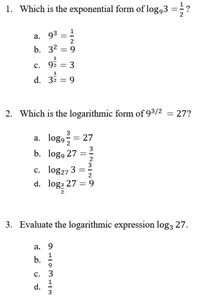Lesson 36 - Definition of Logarithms Quiz

#### 116 Lesson 37 - Properties of Logarithms 17:21

In this lesson we will learn the various properties of logarithms and how to apply them in order to expand or condense a logarithm.

#### 117 Lesson 37 - Properties of Logarithms Answer Key 09:02

Lesson 37 - Properties of Logarithms Answer Key

#### 118 Lesson 37 - Properties of Logarithms Quiz 05:00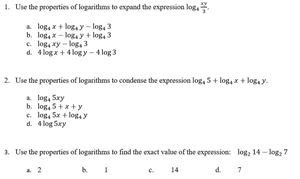Lesson 37 - Properties of Logarithms Quiz

#### 119 Lesson 38 - Common Logs, Natural Logs, and Change of Base 12:05

In this lesson we will learn how to find the exact value of common logarithms, natural logarithms, and change of base.

#### 120 Lesson 38 - Common Logs, Natural Logs, and Change of Base Answer Key 13:53

Lesson 38 - Common Logs, Natural Logs, and Change of Base Answer Key

#### 121 Lesson 38 - Common Logs, Natural Logs, and Change of Base Quiz 10:00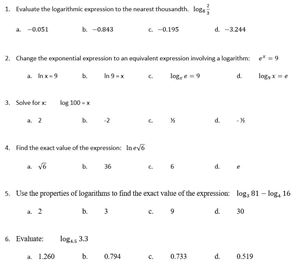Lesson 38 - Common Logs, Natural Logs, and Change of Base Quiz

#### 122 Lesson 39 - Logarithmic Equations Part 1 12:36

In this lesson we will learn how to solve basic logarithmic equations.

#### 123 Lesson 39 - Logarithmic Equations Part 1 Answer Key 06:51

Lesson 39 - Logarithmic Equations Part 1 Answer Key

#### 124 Lesson 39 - Logarithmic Equations Part 1 Quiz 08:00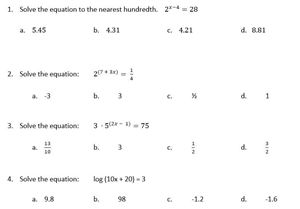Lesson 39 - Logarithmic Equations Part 1 Quiz

#### 125 Lesson 40 - Logarithmic Equations Part 2 12:53

In this lesson we will use our properties of logarithms to help us solve logarithmic equations.

#### 126 Lesson 40 - Logarithmic Equations Part 2 Answer Key 07:42

Lesson 40 - Logarithmic Equations Part 2 Answer Key

#### 127 Lesson 40 - Logarithmic Equations Part 2 Quiz 06:00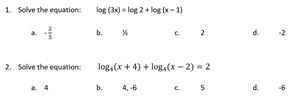Lesson 40 - Logarithmic Equations Part 2 Quiz

#### 128 Lesson 41 - Graphing Logarithms 10:25

In this lesson we will learn how to graph logarithms and find key features including domain, range, intercepts, and an asymptote.

#### 129 Lesson 41 - Graphing Logarithms Answer Keys 08:46

Lesson 41 - Graphing Logarithms Answer Keys

#### 130 Lesson 41 - Graphing Logarithms Quiz 06:00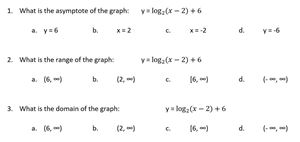Lesson 41 - Graphing Logarithms Quiz

#### 131 Test Unit 7 Lessons 36 - 41 50:00

Test Unit 7 Lessons 36 - 41

#### 132 Lesson 42 - Graphing Polynomials Part 1 15:39

In this lesson we will look at polynomials and determine the degree, the maximum number of turns, and the end behavior based on the degree of the polynomial and the lead coefficient.

#### 133 Lesson 42 - Graphing Polynomials Part 1 Answer Key 05:57

Lesson 42 - Graphing Polynomials Part 1 Answer Key

#### 134 Lesson 42 - Graphing Polynomials Part 1 Quiz 03:00

Lesson 42 - Graphing Polynomials Part 1 Quiz

#### 135 Lesson 43 - Graphing Polynomials Part 2 24:29

In this lesson we will review interval notation, positive versus negative functions, increasing versus decreasing functions, and finding zeros. We will learn how to use this information as well as end behavior to sketch a graph of a polynomial.

#### 136 Lesson 43 - Graphing Polynomials Part 2 Answer Key 13:35

Lesson 43 - Graphing Polynomials Part 2 Answer Key

#### 137 Lesson 43 - Graphing Polynomials Part 2 Quiz 10:00

Lesson 43 - Graphing Polynomials Part 2 Quiz

#### 138 Lesson 44 - Long Division of Polynomials 11:29

In this lesson we will learn how to divide polynomials using long division.

#### 139 Lesson 44 - Long Division of Polynomials Answer Key 14:40

Lesson 44 - Long Division of Polynomials Answer Key

#### 140 Lesson 44 - Long Division of Polynomials Quiz 05:00

Lesson 44 - Long Division of Polynomials Quiz

#### 141 Lesson 45 - Synthetic Division of Polynomials 10:45

In this lesson we will learn how to divide certain types of polynomials using synthetic division.

#### 142 Lesson 45 - Synthetic Division of Polynomials Answer Key 06:33

Lesson 45 - Synthetic Division of Polynomials Answer Key

#### 143 Lesson 45 - Synthetic Division of Polynomials Quiz 07:00

Lesson 45 - Synthetic Division of Polynomials Quiz

#### 144 Lesson 46 - Zeros of a Polynomial Part 1 19:21

In this lesson we will review real zeros including rational and irrational as well as nonreal zeros containing imaginary numbers. We will learn how to find rational zeros and the multiplicity of each.

#### 145 Lesson 46 - Zeros of a Polynomial Part 1 Answer Key 08:05

Lesson 46 - Zeros of a Polynomial Part 1 Answer Key

#### 146 Lesson 46 - Zeros of a Polynomial Part 1 Quiz 05:00

Lesson 46 - Zeros of a Polynomial Part 1 Quiz

#### 147 Lesson 47 - Zeros of a Polynomial Part 2 12:57

In this lesson we will learn to find the equation of a polynomial function if given the degree, the zeros, and/or the end behavior.

#### 148 Lesson 47 - Zeros of a Polynomial Part 2 Answer Key 06:50

Lesson 47 - Zeros of a Polynomial Part 2 Answer Key

#### 149 Lesson 47 - Zeros of a Polynomial Part 2 Quiz 04:00

Lesson 47 - Zeros of a Polynomial Part 2 Quiz

#### 150 Test Unit 8 Lessons 42 - 47 50:00

Test Unit 8 Lessons 42 - 47

#### 151 Lesson 48 - Simplify Rational Expressions 11:33

In this lesson we will learn how to simplify rational expressions.

#### 152 Lesson 48 - Simplify Rational Expressions Answer Key 11:21

Lesson 48 - Simplify Rational Expressions Answer Key

#### 153 Lesson 48 - Simplify Rational Expressions Quiz 07:00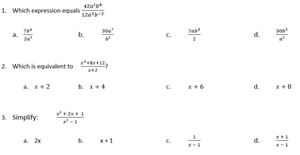Lesson 48 - Simplify Rational Expressions Quiz

#### 154 Lesson 49 - Multiply and Divide Rational Expressions 13:39

In this lesson we will expand on simplifying rational expressions by learning how to multiply and divide rational expressions.

#### 155 Lesson 49 - Multiply and Divide Rational Expressions Answer Key 18:55

Lesson 49 - Multiply and Divide Rational Expressions Answer Key

#### 156 Lesson 49 - Multiply and Divide Rational Expressions Quiz 12:00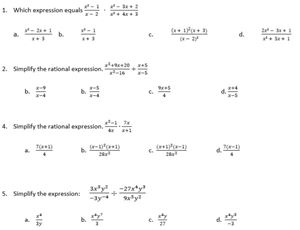Lesson 49 - Multiply and Divide Rational Expressions Quiz

#### 157 Lesson 50 - Add/Subtract Rational Expressions 14:24

In this lesson we will learn how to add or subtract rational expressions.

#### 159 Lesson 50 - Add/Subtract Rational Expressions Quiz 12:00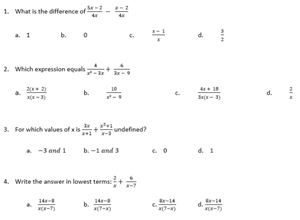Lesson 50 - Add/Subtract Rational Expressions Quiz

#### 160 Lesson 51 - Complex Fractions 12:54

In this lesson we will learn how to simplify a complex fraction.

#### 161 Lesson 51 - Complex Fractions Answer Key 08:53

Lesson 51 - Complex Fractions Answer Key

#### 162 Lesson 51 - Complex Fractions Quiz 08:00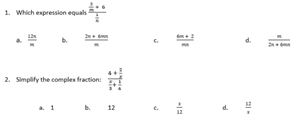Lesson 51 - Complex Fractions Quiz

#### 163 Lesson 52 - Rational Equations Part 1 12:24

In this lesson we will learn how to solve basic rational equations.

#### 164 Lesson 52 - Rational Equations Part 1 Answer Key 07:47

Lesson 52 - Rational Equations Part 1 Answer Key

#### 165 Lesson 52 - Rational Equations Part 1 Quiz 12:00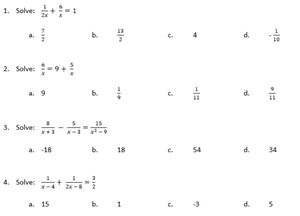Lesson 52 - Rational Equations Part 1 Quiz

#### 166 Lesson 53 - Rational Equations Part 2 11:56

In this lesson we will combine concepts of solving rational equations and solving quadratics.

#### 167 Lesson 53 - Rational Equations Part 2 Answer Keys 10:44

Lesson 53 - Rational Equations Part 2 Answer Keys

#### 168 Lesson 53 - Rational Equations Part 2 Quiz 10:00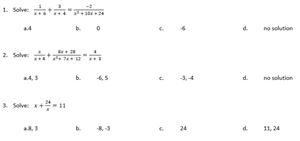Lesson 53 - Rational Equations Part 2 Quiz

#### 169 Lesson 54 - Graphing Rational Functions 24:17

In this lesson we will learn how to find vertical and horizontal asymptotes, and use them with intercepts and test points to graph a rational function.

#### 170 Lesson 54 - Graphing Rational Functions Answer Keys 11:59

Lesson 54 - Graphing Rational Functions Answer Keys

#### 171 Lesson 54 - Graphing Rational Functions Quiz 06:00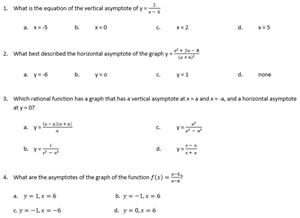Lesson 54 - Graphing Rational Functions Quiz

#### 172 Test Unit 9 Lessons 48 - 54 50:00

Test Unit 9 Lessons 48 - 54

#### 173 Lesson 55 - Graphing Circles 13:45

In this lesson we will learn how to graph by finding the center and radius of a circle. We will work with graphing form and standard form.

#### 174 Lesson 55 - Graphing Circles Answer Keys 11:18

Lesson 55 - Graphing Circles Answer Keys

#### 175 Lesson 55 - Graphing Circles Quiz 05:00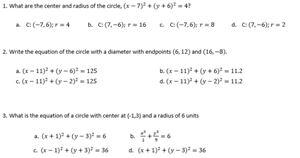Lesson 55 - Graphing Circles Quiz

#### 176 Lesson 56 - Parabolas Part 1 12:53

In this section we will look at graphs of parabolas and find key features such as the vertex, focus, axis of symmetry, directrix, and find the equation.

#### 177 Lesson 56 - Parabolas Part 1 Answer Key 07:23

Lesson 56 - Parabolas Part 1 Answer Key

#### 178 Lesson 56 - Parabolas Part 1 Quiz 10:00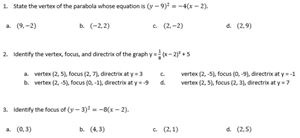Lesson 56 - Parabolas Part 1 Quiz

#### 179 Lesson 57 - Parabolas Part 2 12:13

In this lesson we will be given different key features of a parabola and learn how to find the missing key features and graph.

#### 180 Lesson 57 - Parabolas Part 2 Answer Keys 07:47

Lesson 57 - Parabolas Part 2 Answer Keys

#### 181 Lesson 57 - Parabolas Part 2 Quiz 07:00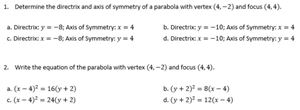Lesson 57 - Parabolas Part 2 Quiz

#### 182 Lesson 58 - Ellipse Part 1 13:57

In this lesson we will learn about an ellipse and the key features to help graph including the center, vertices, co-vertices, and foci.

#### 183 Lesson 58 - Ellipse Part 1 Answer Key 04:30

Lesson 58 - Ellipse Part 1 Answer Key

#### 184 Lesson 58 - Ellipse Part 1 Quiz 06:00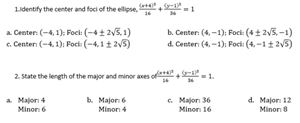Lesson 58 - Ellipse Part 1 Quiz

#### 185 Lesson 59 - Ellipse Part 2 10:50

In this lesson we will be given certain key features of an ellipse and learn to find the rest of the key features.

#### 186 Lesson 59 - Ellipse Part 2 Answer Key 09:57

Lesson 59 - Ellipse Part 2 Answer Key

#### 187 Lesson 59 - Ellipse Part 2 Quiz 10:00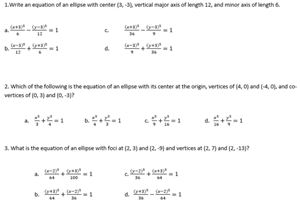Lesson 59 - Ellipse Part 2 Quiz

#### 188 Lesson 60 - Hyperbolas Part 1 16:30

In this lesson we will study hyperbolas and how to find key features including asymptotes, transverse axis, conjugate axis, vertices, foci, and intercepts in order to graph.

#### 189 Lesson 60 - Hyperbolas Part 1 Answer Key 08:34

Lesson 60 - Hyperbolas Part 1 Answer Key

#### 190 Lesson 60 - Hyperbolas Part 1 Quiz 10:00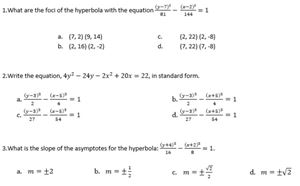Lesson 60 - Hyperbolas Part 1 Quiz

#### 191 Lesson 61 - Hyperbolas Part 2 12:32

In this lesson we will be given specific key features of a hyperbola and we will learn to find the rest of the key features in order to graph.

#### 192 Lesson 61 - Hyperbolas Part 2 Answer Key 13:28

Lesson 61 - Hyperbolas Part 2 Answer Key

#### 193 Lesson 61 - Hyperbolas Part 2 Quiz 06:00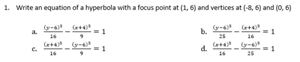Lesson 61 - Hyperbolas Part 2 Quiz

#### 194 Lesson 62 - All Conics 22:31

In this lesson we will be given the equation of a conic. We will learn how to determine which type of conic and then find which key features are necessary to sketch a graph.

#### 195 Lesson 62 - All Conics Answer Key 13:09

Lesson 62 - All Conics Answer Key

#### 196 Lesson 62 - All Conics Quiz 12:00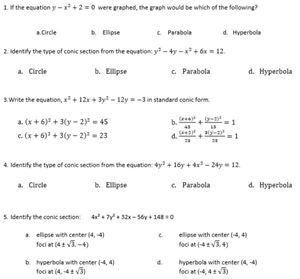Lesson 62 - All Conics Quiz

#### 197 Test Unit 10 Lessons 55 - 62 50:00

Test Unit 10 Lessons 55 - 62

#### 198 Lesson 63 - Sequences Part 1 11:59

In this lesson we will learn about sequences including finite, infinite, arithmetic, geometric, explicit and recursive sequences.

#### 199 Lesson 63 - Sequences Part 1 Answer Keys 14:01

Lesson 63 - Sequences Part 1 Answer Keys

#### 200 Lesson 63 - Sequences Part 1 Quiz 07:00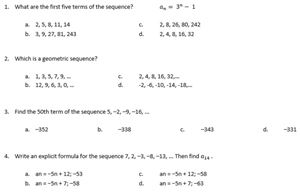Lesson 63 - Sequences Part 1 Quiz

#### 201 Lesson 64 - Sequences Part 2 10:52

In this lesson we will be given specific terms of an arithmetic or geometric sequence and learn how to find any other given term.

#### 202 Lesson 64 - Sequences Part 2 Answer Key 09:19

Lesson 64 - Sequences Part 2 Answer Key

#### 203 Lesson 64 - Sequences Part 2 Quiz 06:00

Lesson 64 - Sequences Part 2 Quiz

#### 204 Lesson 65 - Series Part 1 11:49

In this lesson we will learn about series and sigma notation.

#### 205 Lesson 65 - Series Part 1 Answer Key 04:17

Lesson 65 - Series Part 1 Answer Key

#### 206 Lesson 65 - Series Part 1 Quiz 06:00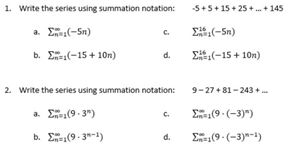Lesson 65 - Series Part 1 Quiz

#### 207 Lesson 66 - Series Part 2 09:49

In this lesson we will learn to find the partial sum of an arithmetic sequence, the partial sum of a geometric sequence, and the infinite sum of a geometric sequence.

#### 208 Lesson 66 - Series Part 2 Answer Keys 10:35

Lesson 66 - Series Part 2 Answer Keys

#### 209 Lesson 66 - Series Part 2 Quiz 08:00

Lesson 66 - Series Part 2 Quiz

#### 210 Test Unit 11 Lessons 63 - 66 50:00

Test Unit 11 Lessons 63 - 66

Course Overview

This “Math Grade 11” video course covers all content areas students need to successfully complete an Algebra 2 course. This course has an emphasis on combining multiple concepts and using critical thinking skills. This course reviews necessary skills from Algebra 1 and Geometry while preparing students who may take a Trigonometry or College Algebra class. This is a full year’s 11th Grade Math Algebra 2 course! This course can also be taken by students in grade 10.

This Course Includes:

• 11 units

• 85 Worksheets with counterpart answer keys

• 66 video-lessons

• 67 exercise review videos (I explain step by step how to reach the correct answer)

• 66 online quizzes

• Over 20 hours of video lessons!

Course Goals

Upon completion of this course, students will be able to successfully work with different concept areas including but not limited to inequalities, absolute values, systems of equations, graphing, radicals, matrices, logarithms, quadratics, polynomials, parabolas, circles, hyperbolas, ellipses, sequences and series.

Target Audience

This video-course is primarily intended for students who are interested in learning a typical Algebra 2 course and have successfully completed Algebra 1 and Geometry.

Course Requirements

Students taking this course will need to have completed Math Grades 9 and 10 also known as Algebra 1 and Geometry.

Course Topics

Unit 1: Inequalities, Absolute Value Equations, Absolute Value Inequalities, General Problem Solving

Unit 2: Graphing Parent Functions and Transformations, Graph Reading, Graphing Piecewise Functions, Functions, Composite Functions, Inverse Functions

Unit 3: Solving systems in 2 variables, Solving systems in 3 variables, Systems of Inequalities, Linear Programming

Unit 4: Basic Matrices, Multiplying Matrices, Determinants, Inverse Matrices, Matrix Equations

Unit 7: Definition of Logarithms, Properties of Logarithms, Common Logarithms, Natural Logarithms, Change of Base, Logarithmic Equations, Graphing Logarithms

Unit 8: Graphing Polynomials, Long Division of Polynomials, Synthetic Division of Polynomials, Zeros of a Polynomial

Unit 9: Simplify Rational Expressions, Multiple/Divide Rational Expressions, Add/Subtract Rational Expressions, Complex Fractions, Rational Equations, Graphing Rational Functions

Unit 10: Circles, Parabolas, Ellipses, Hyperbolas

Unit 11: Sequences, Series

• Teacher: Catherine
• Areas of expertise: High School Mathematics
• Education: Bachelor's Degree in Mathematics Master's Degree in Education
• Interests: I enjoy spending time with my family. We especially enjoy outdoor adventures and being active.
• Skills: I am able to explain complicated mathematical concepts with ease.
• Associations: Illinois Teaching Certificate
• Issues I care about: I believe all people should have the opportunity to an equal education. I know that our youth is our future and I want to ensure they have the tools and thinking skills necessary to make a positive difference.

I have always enjoyed math and really found my calling to it in high school. I want students to see that math can be a fun puzzle to solve. I have taught various levels of Algebra 1, Geometry, Algebra 2, and Trigonometry/Statistics in my 8 years teaching high school math. Through my experience with teaching and tutoring, I am able to know what questions students will have and explain them throughout the lesson. I ensure to review previously learned math material needed to be successful in each lesson. I look forward to helping you learn Algebra 2!

#### Digital Quizzes and Tests - Answer Keys

Digital Quizzes and Tests - Answer Keys

#### Test Preparation Document

Test Preparation Document

#### Digital Quizzes and Tests Answer Keys Document

Digital Quizzes and Tests Answer Keys Document

#### Test Unit 1 Lessons 1 - 4 Answer Key

Test Unit 1 Lessons 1 - 4 Answer Key

#### Test Unit 2 Lessons 5 - 11 Answer Key

Test Unit 2 Lessons 5 - 11 Answer Key

#### Test Unit 3 Lessons 12 - 15 Answer Key

Test Unit 3 Lessons 12 - 15 Answer Key

#### Test Unit 4 Lessons 16 - 20 Answer Key

Test Unit 4 Lessons 16 - 20 Answer Key

#### Test Unit 5 Lessons 21 - 27 Answer Key

Test Unit 5 Lessons 21 - 27 Answer Key

#### Test Unit 6 Lessons 28 - 35 Answer Key

Test Unit 6 Lessons 28 - 35 Answer Key

#### Test Unit 7 Lessons 36 - 41 Answer Key

Test Unit 7 Lessons 36 - 41 Answer Key

#### Test Unit 8 Lessons 42 - 47 Answer Key

Test Unit 8 Lessons 42 - 47 Answer Key

#### Test Unit 9 Lessons 48 - 54 Answer Key

Test Unit 9 Lessons 48 - 54 Answer Key

#### Test Unit 10 Lessons 55 - 62 Answer Key

Test Unit 10 Lessons 55 - 62 Answer Key

#### Test Unit 11 Lessons 63 - 66 Answer Key

Test Unit 11 Lessons 63 - 66 Answer Key
Customers who bought this item also boughtThis is a rich and exciting course that is foundational to all other science courses. In fact, chemistry is often called "The Central Science". We will learn about atoms, molecules, acids, chemical reactions and equations and much more! +
\$249.99 \$104.00This U.S. History course begins with an introduction to historical eras and then covers America's founding documents before progressing chronologically through the major eras in history beginning with the U.S. Civil War and Reconstruction through the decade of the 2010s. +
\$299.00 \$179.00Take a dive into the inner workings of your brain and human behavior! This course has everything you need to succeed! Learn about how psychologists conduct research, how the brain and your senses organize and interpret information, how we learn and remember (and forget). See you soon! +
\$249.00 \$104.00